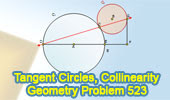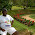## Wednesday, September 29, 2010

### Problem 523: Tangent Circles, Diameter Perpendicular, Collinearity

Geometry Problem
Level: High School, SAT Prep, College geometry.
Click the figure below to see the complete problem 523 about Tangent Circles, Diameter Perpendicular, Collinearity.#### 4 comments:

1.BGD = ADG ( alternate ang )
BGC = BCG, ADC = ACD
=>
ACD = BCG
it happen when "they" are verticales angles
or D, C, G are collinear

2.Note that points A, C and B are collinear ( Circle C1 tangent to C2 at C)
BG perpendicular to GF ( FG tangent to C2 at G)
So DF // BG and angle DAC=angle CBG ( alternate angles)
And angle ACD= angle BCG ( Isosceles triangles DAC and CBG are similar)
So Points D, C G are collinear

Peter Tran

3.(Proof of Contradiction)
Connect CE and draw common tangent at E (intersect the tangent at EF and call the intersection H).
Suppose if DCG are noncollinear, then there's Quadrilateral CDFG. Angle DCE is right angle (inscribed angle on diameter), Angle ECH=Angle CDE (tangent angle equal to inscribed angle corresponding to its intercepted arc), Angle GCH=Angle CGF (transveral connecting two tangent points have equal consecutive interior angles).
Add up the angles in Quadrilateral CDFG, Angles (ECH+DCE+CDE+GCH+CGF+DFG)=360 Degrees.
But since DFG (given) and DCE are both right angles, Angle ECH=Angle CDE, and Angle GCH=Angle CGF, this time Angles 2(DCE+ECH+GCH)=2 Angle DCG=360 Degrees, so Angle DCG=180 Degrees, supposing a quadrilateral--contradiction!
Therefore, DCG are collinear.

4.Let the tangents at C and G to circle B meet at H. Now HC is perpendicular to ACB. Hence HCBG & ACFH are both cyclic. So < DCA = 1/2 < CAE = 1/2 < CHF = supplementary of B / 2 = < BCG.

Hence DBG are collinear

Sumith Peiris
Moratuwa
Sri Lanka